# Relationship between Yarn Count and Twist | Principles of Twist Measuring Methods

Last Updated on 13/06/2021

### Yarn Twist: Types, Factors and Measuring Methods

Md. Jasimuddin Mandal
Govt. College of Engineering and Textile Technology,
Serampore, India.
Email ID: jasimmandal@gmail.com

Yarn Twist:
In the manufacturing of staple fiber yarns, twist is inserted into the fine strand of fibers to hold the fibers together and impart the desired properties to the twisted yarns. Without twist, the fine strand of fibers would be very weak and of little practical use. A change in the level of twist also changes many yarn properties, such as strength and softness.

Definition of Twist:
Twist may be defined as the spiral disposition of the components of a thread which is usually the result of relative rotation of the two ends. Twist is generally expressed as the number of turns per unit length of yarn, e.g. turns per inch (tpi), turns per metre (tpm), etc. The traditional staple yarns are strengthened by means of twisting the bundle of fibers. The number of coils inserted per unit length of yarn is called twist Z. This is expressed as,

.. ….Nc
Z = ——–
..  ….l

Where, Nc denotes the number of coils inserted in a length l of yarn. Its dimension is usually expressed in m–1 or inch–1.

What exactly does twist to a yarn?

1. The twist in a yarn binds the fibers together and helps to keep them in the respective positions. It thus gives coherence to yarn.
2. Twist gives sufficient strength to the yarn.
3. Twisting is also used to bring about novel effects that are prominently visible when the yarn is converted to fabric. This is achieved primarily by having a combination of yarns with different twist levels and twist directions in the fabric.

Twist Intensity
The quantity DZ is therefore dimensionless. Nevertheless, we usually use the following dimensionless quantity:

κ = πDZ

Where κ is called twist intensity. The geometrical sense of twist intensity is depicted in below Figure.

The cylinder, shown in Figure-1(a), symbolizes a portion of a yarn of length 1/Z . It contains only one coil of ideal fibers. The peripheral fibers are lying at the cylindrical surface of the yarn, i.e., at yarn diameter D. We assume that these fibers follow the helical shape. The angle βD is defined as an angle between the tangent to the fiber helix and the direction of yarn axis.

Unrolling of the cylindrical surface would result in a triangle, shown in Figure-1(b). It is then valid to write that

tanβD = πD (1/Z ) = πDZ = κ

So, the twist intensity represents the tangent of angle βD of the peripheral fibers in a yarn. This variable is evidently dimensionless.

Nature of Twist:

Types of Twist:
There are two types of twist:

1. Real twist and
2. False twist.

1. Real twist:

• To insert a real twist into a length of yarn, one end of the yarn should be rotated relative to the other end, as indicated in figure (a).
• Spun yarns usually have real twist, which holds the fibers together in the yarn.

2. False twist:

• When inserting false twist into a length of yarn, both ends of the yarn are clamped, usually by rollers, and twist is inserted with a false twister between the clamping points, as indicated in Figure-2(b).
• If the yarn is not traversing along its axis, the twist will be in opposite directions above and below the false twister. If the false twister is removed, the opposite twists will cancel out one another, leaving no real twist in the length of yarn. If the yarn is traversing along its axis, then the section of the yarn moving away from the false twister would have no net twist, as indicated in Figure-2(b).
• False twisting is a very important phenomenon, which has considerable practical implications in yarn technology.

Twist Direction:
A twist can be either in Z direction or S direction as indicated in the following figure, depending on the orientation of the surface fiber in relation to yarn axis.

It is worth noting that twist direction affects fabric properties. For example, following Figure shows two identical twill-weave fabrics with the warp yarn of different twist direction. Fabric A will be more lustrous than fabric B, because light reflected by fibers in the warp and weft is in the same direction. Fabric A will be softer while fabric B firmer, because in Fabric B, the surface fibers on the warp and weft in the region of contact are aligned in the same direction and they may ‘get stuck’ inside each other and reduce the mobility of the intersection. Whereas for fabric A, the surface fibers on the warp and weft in the region of contact are crossed over, and they can move about easily. The freedom of movement at the yarn intersections is the key for fabric softness.

Self-locking Effect:
Because of twist in a yarn, the fibers on yarn surface take a roughly helical configuration around the yarn. When the yarn is under tension, these surface fibers are also under tension. However, because of the helical configuration, part of the tension is diverted radially, which creates a radial pressure. This is illustrated in the following figure.

The radial pressure tends to pack the fibers together, increasing the normal force between them, and so increasing their frictional resistance to slipping past each other. The more tension is applied to the yarn, the more it locks together, hence ‘self-locking’. An analogy is, when you wind a string around your arm, as you pull the string along the arm and away from each other, the string bites deeper into the flesh.

Without twist, there won’t be any self-locking effect to prevent fiber slippage. Consequently the yarn would have no strength.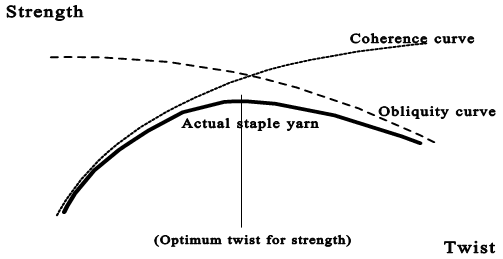Relationship between Yarn Count and Twist:From figure, we get,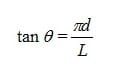(Where, ɵ= twist angle, d= yarn diameter and L= yarn length)

Also from figure, the height (pitch) of one turn of twist is L. Since the twist level is normally specified as the number of turns per metre, the twist level in one meter of the yarn would be: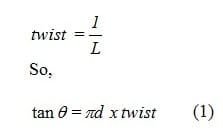We also know from experience that yarn diameter is also very hard to measure, because textile yarns by their very nature are soft and squashy. On the other hand, yarn count is normally used as we have discussed in the first topic of this module. But we can relate yarn diameter to yarn count using the expression below:

Cubic density (ρ) = linear density (Tex) / cross-sectional area (A)

Assuming a circular cross section for the yarn, we get,K is called the twist factor, and is proportional to θ if ρ remains constant.

Thus, K is a factor relating twisting level to yarn count. The derivation shows that if two yarns have the same twist factor, they will have the same surface twist angle, regardless of count. Since surface twist angle is the main factor determining yarn character, then twist factor can be used to define the character of a yarn.

It is worth noting though there are minor errors associated with the use of twist factor for the following reasons:

• The cubic density may be different for different yarns. It is assumed in the above calculation that this will not change for yarns of the same surface twist angle.
• Different fibers with different frictional and other properties will create different yarn character.

Nevertheless, the relationship we have just derived between twist, twist factor and yarn count is one of the most important in the study of yarn technology. This relationship is expressed in different ways for different yarn counting systems.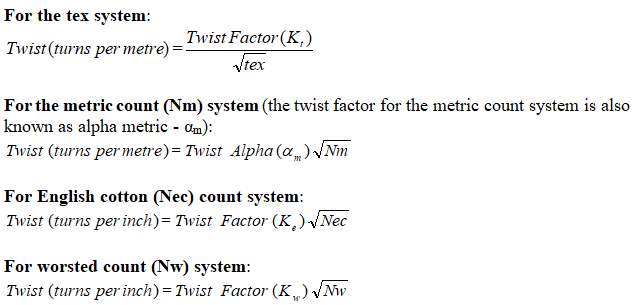Please note the unit for twist is also different in the above expressions of twist factor. In addition, twist factor is also known as twist multiplier, twist alpha, or twist coefficient.

Angle of Twist:
The yarn twist angle is the angle between a tangent to the helix formed by a fiber on the yarn surface and the yarn axis. If the twist multiplier of a cotton yarn is known, the twist angle can be easily calculated.

Factors Affecting of Yarn Twist:
The twist introduced in the yarn during spinning depends upon a number of factors, such as follows:

1. The count of yarn to be spun
2. The quality of cotton used
3. The use to which the yarn is put- is the yarn meant to be used as warp yarn or weft yarn, knitting yarn or any other yarn?
4. The fineness of the fiber being spun
5. The softness of the fabric into which the yarn is to be converted

The Distribution of Twist in Staple Spun Yarns:
If someone twists your head, it is your neck that suffers most. That is because the neck is a ‘thin’ place and offers little resistance to being twisted. By analogy, if a yarn of varying thickness is twisted, it is usually the thin spot in the yarn that gets twisted the most. Invariably, yarns spun from staple fibers (e.g. wool, cotton) are not perfectly uniform, and there are thick and thin spots along the yarn length. This variation in yarn thickness will lead to variation in the twist level along the yarn length, because twist tends to accumulate in the thin place.

The fact that twist tends to accumulate in the thin spot along the yarn has several important implications:

1. It exacerbates the variation in yarn linear density
While variation in yarn linear density is the fundamental cause of twist variation, concentration of twist in the thin places will make those places even thinner, exacerbating the problem of yarn unevenness.

2. It improves the evenness of a fiber assembly during “drafting against twist”
In the drafting stage of woollen ring spinning, the woollen slubbing is drafted while twist is inserted into the slubbing (drafting against twist) to control fibers during drafting. Because twist tends to accumulate in the thin spots, the fibers in thin regions in the slubbing are more difficult to draft than those in the thick places, which have less twist. As a result, the thick places are drafted more than the thin places, thus improving the evenness of the drafted material. This is depicted in following figure.

3. It has implication for twist measurements
Because the twist level varies along the yarn length, the twist measured at a short length of yarn may not reflect the true average twisting of the yarn. Standard test procedures should be followed to measure the yarn twist accurately.

The relationship between twist and yarn count may be expressed by the following formula:Where, p is usually greater than 1 but less than 2 for most yarns.

Twist Contraction:
When a bundle of parallel fibers is twisted, the distance between the two ends of a fiber will decrease, particularly for fibers near the surface of the twisted bundle. As a result, the overall length of the twisted bundle is shorter than its length before twist insertion. The reduction in length due to twist insertion is known as twist contraction.

The following formula is used to calculate the amount of twist contraction: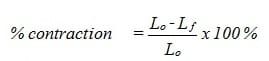Where,
Lo = original length before twisting
Lf = final length after twisting

It should be noted that because of twist contraction and the associated change in length, the count of a yarn will change slightly when twist in the yarn is changed. Twist contraction increases yarn count (tex), because the weight of the yarn is distributed over a shorter length. The following formula can be usedWhere,
No = count (tex) before twisting
Nf = count (tex) after twisting
C = %contraction

Measurement of Twist:
Twist measurement is a routine test for yarns. Because of the variation in twist along yarn length, care should be taken in measuring the twist of staple spun yarns. Some basic principles are discussed here.

Sampling Rules:
The following rules should be observed when measuring yarn twist:

1. Tests should not be limited to a short length of the yarn package.
2. Beware of “operator bias” – tendency to select either thicker or thinner regions. Taking samples at fixed intervals along the yarn length will reduce the bias.
3. Discard first few meters from package. Being a free end, it could have lost twist.
4. Remove yarn from side of package, not over end. Removing yarn over end will change the twist level in the yarn.
5. Tension in Yarn during test e.g. for single worsted yarns: 5 + 1 mN/tex

Principles of Twist Measuring Methods:
The two common methods used in twist measurement are straightened fiber method and untwist/retwist method.

(1) Straightened Fiber Method:
This method involves counting of the number of turns required to untwist the yarns until the surface fibers appear to be straight and parallel to yarn axis. This method is mainly used for ply and continuous filament yarns.

(2) Untwist / Retwist Method:
This is the common method used for staple fiber yarns. It is based on twist contraction (hence also known as twist contraction method).

For this method, it is assumed that the contraction in length, due to insertion of twist, is the same for both direction of twist (S and Z). Suppose we want to measure the twist level in a yarn with Z twist, the yarn is first untwisted (by a twist tester), and a counter on the twist tester will record the number of turns. During untwisting, the yarn would increase in length from its original length L to a new length L’. If the operation is continued, the yarn would have its twist completely removed first and then twisted up again in S direction. As the yarn gets twisted, its length will decrease (twist contraction) from L’ towards its original length L. When its original length is reached, the total number of turns received by the yarn, as recorded by the counter on the twist tester, would be equal to twice the twist in the original yarn (with a length of L).

Automatic twist testers are now available, such as the Zweigle automatic twist tester.

References:

1. Principles of Textile Testing by J.E. Booth
2. Theory of Structure and Mechanics of Yarns by Bohuslav Neckář and Dipayan Das
3. Hearle, J. W. S.; Grosberg, P.; Backer, S. Structural Mechanics of Yarns and Fabrics; Wiley-Interscience: New York, 1969; Vol. 1
4. Textile testingI- NCUTE

You may also like:

1.